# Head model and forward computation#

The aim of this tutorial is to be a getting started for forward computation.

For more extensive details and presentation of the general concepts for forward modeling, see The forward solution.

```import mne
from mne.datasets import sample

data_path = sample.data_path()

# the raw file containing the channel location + types
sample_dir = data_path / "MEG" / "sample"
raw_fname = sample_dir / "sample_audvis_raw.fif"
# The paths to Freesurfer reconstructions
subjects_dir = data_path / "subjects"
subject = "sample"
```

## Computing the forward operator#

To compute a forward operator we need:

• a `-trans.fif` file that contains the coregistration info.

• a source space

• the BEM surfaces

## Compute and visualize BEM surfaces#

The BEM surfaces are the triangulations of the interfaces between different tissues needed for forward computation. These surfaces are for example the inner skull surface, the outer skull surface and the outer skin surface, a.k.a. scalp surface.

Computing the BEM surfaces requires FreeSurfer and makes use of the command-line tools mne watershed_bem or mne flash_bem, or the related functions `mne.bem.make_watershed_bem()` or `mne.bem.make_flash_bem()`.

Here we’ll assume it’s already computed. It takes a few minutes per subject.

For EEG we use 3 layers (inner skull, outer skull, and skin) while for MEG 1 layer (inner skull) is enough.

Let’s look at these surfaces. The function `mne.viz.plot_bem()` assumes that you have the `bem` folder of your subject’s FreeSurfer reconstruction, containing the necessary surface files. Here we use a smaller than default subset of `slices` for speed.

```plot_bem_kwargs = dict(
subject=subject,
subjects_dir=subjects_dir,
brain_surfaces="white",
orientation="coronal",
slices=[50, 100, 150, 200],
)

mne.viz.plot_bem(**plot_bem_kwargs)
```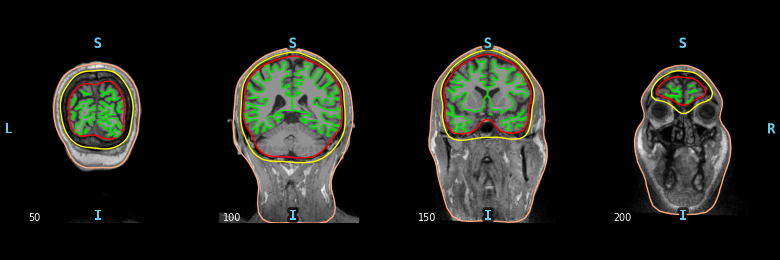```Using surface: /home/circleci/mne_data/MNE-sample-data/subjects/sample/bem/inner_skull.surf
Using surface: /home/circleci/mne_data/MNE-sample-data/subjects/sample/bem/outer_skull.surf
Using surface: /home/circleci/mne_data/MNE-sample-data/subjects/sample/bem/outer_skin.surf
```

## Visualizing the coregistration#

The coregistration is the operation that allows to position the head and the sensors in a common coordinate system. In the MNE software the transformation to align the head and the sensors in stored in a so-called trans file. It is a FIF file that ends with `-trans.fif`. It can be obtained with `mne.gui.coregistration()` (or its convenient command line equivalent mne coreg), or mrilab if you’re using a Neuromag system.

Here we assume the coregistration is done, so we just visually check the alignment with the following code.

```# The transformation file obtained by coregistration
trans = sample_dir / "sample_audvis_raw-trans.fif"

# Here we look at the dense head, which isn't used for BEM computations but
# is useful for coregistration.
mne.viz.plot_alignment(
info,
trans,
subject=subject,
dig=True,
meg=["helmet", "sensors"],
subjects_dir=subjects_dir,
)
```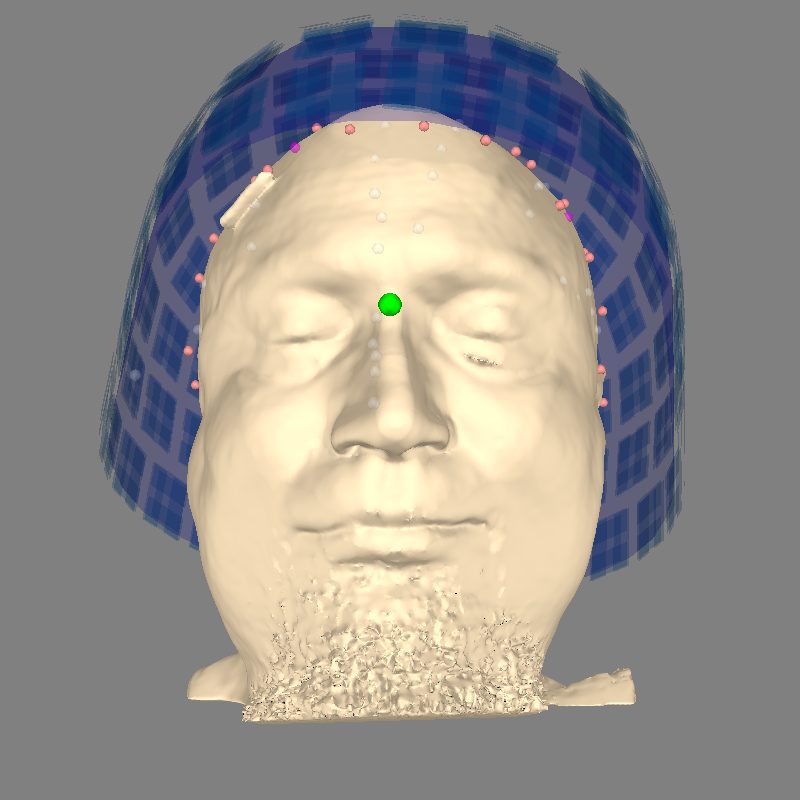```    Read a total of 3 projection items:
PCA-v1 (1 x 102)  idle
PCA-v2 (1 x 102)  idle
PCA-v3 (1 x 102)  idle
Getting helmet for system 306m
Channel types:: grad: 203, mag: 102, eeg: 59
```

## Compute Source Space#

The source space defines the position and orientation of the candidate source locations. There are two types of source spaces:

• surface-based source space when the candidates are confined to a surface.

• volumetric or discrete source space when the candidates are discrete, arbitrarily located source points bounded by the surface.

Surface-based source space is computed using `mne.setup_source_space()`, while volumetric source space is computed using `mne.setup_volume_source_space()`.

We will now compute a surface-based source space with an `'oct4'` resolution. See Setting up the source space for details on source space definition and spacing parameter.

Warning

`'oct4'` is used here just for speed, for real analyses the recommended spacing is `'oct6'`.

```src = mne.setup_source_space(
)
print(src)
```
```Setting up the source space with the following parameters:

SUBJECTS_DIR = /home/circleci/mne_data/MNE-sample-data/subjects
Subject      = sample
Surface      = white

>>> 1. Creating the source space...

Doing the octahedral vertex picking...
Mapping lh sample -> oct (4) ...
Triangle neighbors and vertex normals...
Setting up the triangulation for the decimated surface...
loaded lh.white 258/155407 selected to source space (oct = 4)

Mapping rh sample -> oct (4) ...
Triangle neighbors and vertex normals...
Setting up the triangulation for the decimated surface...
loaded rh.white 258/156866 selected to source space (oct = 4)

Calculating patch information (limit=0.0 mm)...
Computing patch statistics...
Computing patch statistics...
You are now one step closer to computing the gain matrix
<SourceSpaces: [<surface (lh), n_vertices=155407, n_used=258>, <surface (rh), n_vertices=156866, n_used=258>] MRI (surface RAS) coords, subject 'sample', ~28.7 MB>
```

The surface based source space `src` contains two parts, one for the left hemisphere (258 locations) and one for the right hemisphere (258 locations). Sources can be visualized on top of the BEM surfaces in purple.

```mne.viz.plot_bem(src=src, **plot_bem_kwargs)
```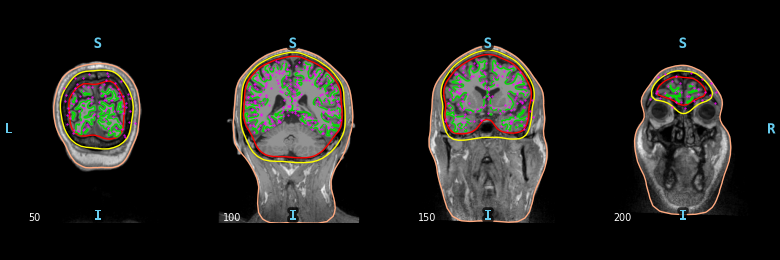```Using surface: /home/circleci/mne_data/MNE-sample-data/subjects/sample/bem/inner_skull.surf
Using surface: /home/circleci/mne_data/MNE-sample-data/subjects/sample/bem/outer_skull.surf
Using surface: /home/circleci/mne_data/MNE-sample-data/subjects/sample/bem/outer_skin.surf
```

To compute a volume based source space defined with a grid of candidate dipoles inside a sphere of radius 90mm centered at (0.0, 0.0, 40.0) mm you can use the following code. Obviously here, the sphere is not perfect. It is not restricted to the brain and it can miss some parts of the cortex.

```sphere = (0.0, 0.0, 0.04, 0.09)
vol_src = mne.setup_volume_source_space(
subject,
subjects_dir=subjects_dir,
sphere=sphere,
sphere_units="m",
)  # just for speed!
print(vol_src)

mne.viz.plot_bem(src=vol_src, **plot_bem_kwargs)
```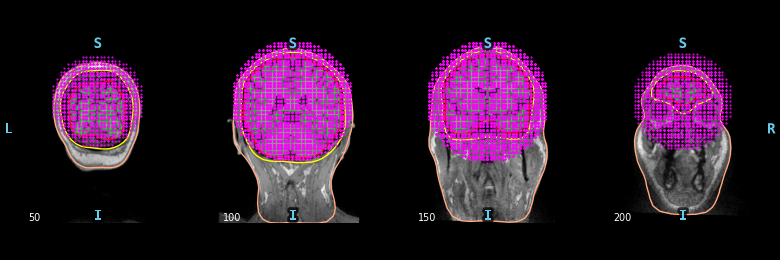```Sphere                : origin at (0.0 0.0 40.0) mm
grid                  : 5.0 mm
mindist               : 5.0 mm
MRI volume            : /home/circleci/mne_data/MNE-sample-data/subjects/sample/mri/T1.mgz

Setting up the sphere...
Surface CM = (   0.0    0.0   40.0) mm
Surface fits inside a sphere with radius   90.0 mm
Surface extent:
x =  -90.0 ...   90.0 mm
y =  -90.0 ...   90.0 mm
z =  -50.0 ...  130.0 mm
Grid extent:
x =  -95.0 ...   95.0 mm
y =  -95.0 ...   95.0 mm
z =  -50.0 ...  135.0 mm
57798 sources before omitting any.
24365 sources after omitting infeasible sources not within 0.0 - 90.0 mm.
20377 sources remaining after excluding the sources outside the surface and less than    5.0 mm inside.
Source space : MRI voxel -> MRI (surface RAS)
0.005000  0.000000  0.000000     -95.00 mm
0.000000  0.005000  0.000000     -95.00 mm
0.000000  0.000000  0.005000     -50.00 mm
0.000000  0.000000  0.000000       1.00
MRI volume : MRI voxel -> MRI (surface RAS)
-0.001000  0.000000  0.000000     128.00 mm
0.000000  0.000000  0.001000    -128.00 mm
0.000000 -0.001000  0.000000     128.00 mm
0.000000  0.000000  0.000000       1.00
MRI volume : MRI (surface RAS) -> RAS (non-zero origin)
1.000000 -0.000000 -0.000000      -5.27 mm
-0.000000  1.000000 -0.000000       9.04 mm
-0.000000  0.000000  1.000000     -27.29 mm
0.000000  0.000000  0.000000       1.00
<SourceSpaces: [<volume, shape=(39, 39, 38), n_used=20377>] MRI (surface RAS) coords, subject 'sample', ~14.3 MB>
Using surface: /home/circleci/mne_data/MNE-sample-data/subjects/sample/bem/inner_skull.surf
Using surface: /home/circleci/mne_data/MNE-sample-data/subjects/sample/bem/outer_skull.surf
Using surface: /home/circleci/mne_data/MNE-sample-data/subjects/sample/bem/outer_skin.surf
```

To compute a volume based source space defined with a grid of candidate dipoles inside the brain (requires the BEM surfaces) you can use the following.

```surface = subjects_dir / subject / "bem" / "inner_skull.surf"
vol_src = mne.setup_volume_source_space(
)  # Just for speed!
print(vol_src)

mne.viz.plot_bem(src=vol_src, **plot_bem_kwargs)
```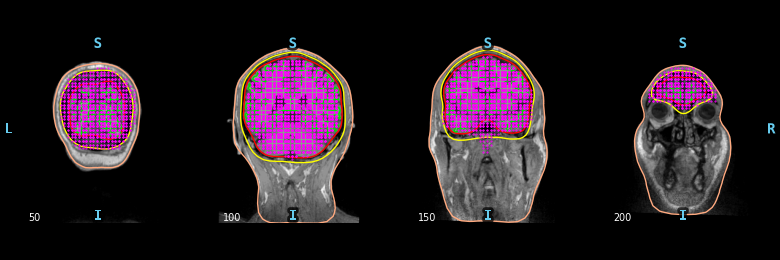```Boundary surface file : /home/circleci/mne_data/MNE-sample-data/subjects/sample/bem/inner_skull.surf
grid                  : 5.0 mm
mindist               : 5.0 mm
MRI volume            : /home/circleci/mne_data/MNE-sample-data/subjects/sample/mri/T1.mgz

Loaded bounding surface from /home/circleci/mne_data/MNE-sample-data/subjects/sample/bem/inner_skull.surf (2562 nodes)
Surface CM = (   0.7  -10.0   44.3) mm
Surface fits inside a sphere with radius   91.8 mm
Surface extent:
x =  -66.7 ...   68.8 mm
y =  -88.0 ...   79.0 mm
z =  -44.5 ...  105.8 mm
Grid extent:
x =  -70.0 ...   70.0 mm
y =  -90.0 ...   80.0 mm
z =  -45.0 ...  110.0 mm
32480 sources before omitting any.
22941 sources after omitting infeasible sources not within 0.0 - 91.8 mm.
Source spaces are in MRI coordinates.
Checking that the sources are inside the surface and at least    5.0 mm away (will take a few...)
Checking surface interior status for 22941 points...
Found  2787/22941 points inside  an interior sphere of radius   43.6 mm
Found     0/22941 points outside an exterior sphere of radius   91.8 mm
Found  9357/20154 points outside using surface Qhull
[Parallel(n_jobs=1)]: Using backend SequentialBackend with 1 concurrent workers.
[Parallel(n_jobs=1)]: Done   1 out of   1 | elapsed:   13.9s remaining:    0.0s
[Parallel(n_jobs=1)]: Done   1 out of   1 | elapsed:   13.9s finished
Found   846/10797 points outside using solid angles
Total 12738/22941 points inside the surface
Interior check completed in 13892.1 ms
10203 source space points omitted because they are outside the inner skull surface.
2362 source space points omitted because of the    5.0-mm distance limit.
10376 sources remaining after excluding the sources outside the surface and less than    5.0 mm inside.
Source space : MRI voxel -> MRI (surface RAS)
0.005000  0.000000  0.000000     -70.00 mm
0.000000  0.005000  0.000000     -90.00 mm
0.000000  0.000000  0.005000     -45.00 mm
0.000000  0.000000  0.000000       1.00
MRI volume : MRI voxel -> MRI (surface RAS)
-0.001000  0.000000  0.000000     128.00 mm
0.000000  0.000000  0.001000    -128.00 mm
0.000000 -0.001000  0.000000     128.00 mm
0.000000  0.000000  0.000000       1.00
MRI volume : MRI (surface RAS) -> RAS (non-zero origin)
1.000000 -0.000000 -0.000000      -5.27 mm
-0.000000  1.000000 -0.000000       9.04 mm
-0.000000  0.000000  1.000000     -27.29 mm
0.000000  0.000000  0.000000       1.00
<SourceSpaces: [<volume, shape=(29, 35, 32), n_used=10376>] MRI (surface RAS) coords, subject 'sample', ~8.0 MB>
Using surface: /home/circleci/mne_data/MNE-sample-data/subjects/sample/bem/inner_skull.surf
Using surface: /home/circleci/mne_data/MNE-sample-data/subjects/sample/bem/outer_skull.surf
Using surface: /home/circleci/mne_data/MNE-sample-data/subjects/sample/bem/outer_skin.surf
```

Note

Some sources may appear to be outside the BEM inner skull contour. This is because the `slices` are decimated for plotting here. Each slice in the figure actually represents several MRI slices, but only the MRI voxels and BEM boundaries for a single (midpoint of the given slice range) slice are shown, whereas the source space points plotted on that midpoint slice consist of all points for which that slice (out of all slices shown) was the closest.

Now let’s see how to view all sources in 3D.

```fig = mne.viz.plot_alignment(
subject=subject,
subjects_dir=subjects_dir,
surfaces="white",
coord_frame="mri",
src=src,
)
mne.viz.set_3d_view(
fig,
azimuth=173.78,
elevation=101.75,
distance=0.30,
focalpoint=(-0.03, -0.01, 0.03),
)
```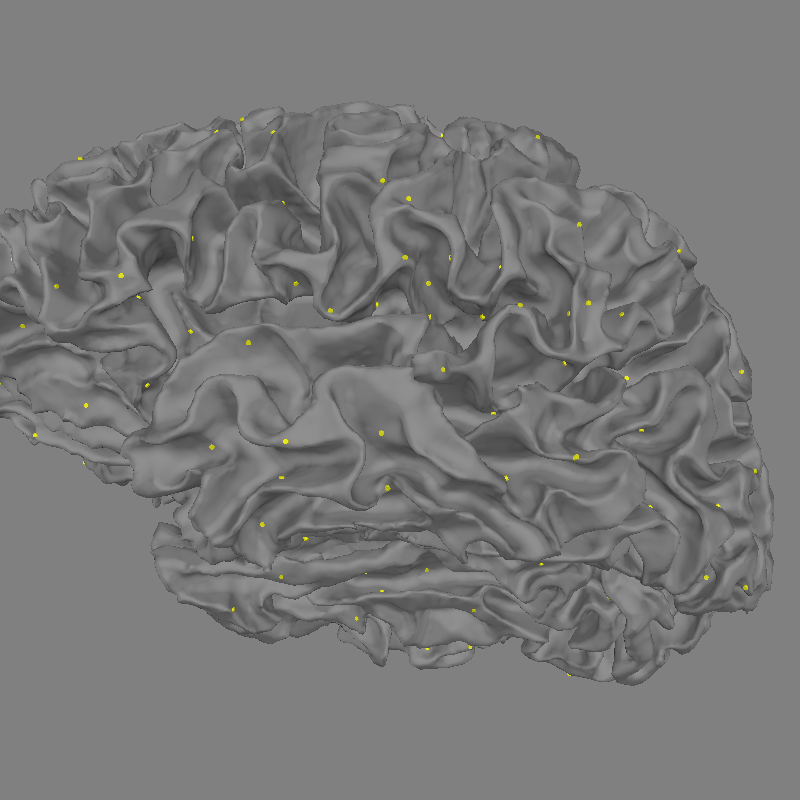## Compute forward solution#

We can now compute the forward solution. To reduce computation we’ll just compute a single layer BEM (just inner skull) that can then be used for MEG (not EEG). We specify if we want a one-layer or a three-layer BEM using the `conductivity` parameter. The BEM solution requires a BEM model which describes the geometry of the head the conductivities of the different tissues.

```conductivity = (0.3,)  # for single layer
# conductivity = (0.3, 0.006, 0.3)  # for three layers
model = mne.make_bem_model(
subject="sample", ico=4, conductivity=conductivity, subjects_dir=subjects_dir
)
bem = mne.make_bem_solution(model)
```
```Creating the BEM geometry...
Going from 4th to 4th subdivision of an icosahedron (n_tri: 5120 -> 5120)
inner skull CM is   0.67 -10.01  44.26 mm
Surfaces passed the basic topology checks.
Complete.

Computing the linear collocation solution...
Matrix coefficients...
inner skull (2562) -> inner skull (2562) ...
Inverting the coefficient matrix...
BEM geometry computations complete.
```

Note that the BEM does not involve any use of the trans file. The BEM only depends on the head geometry and conductivities. It is therefore independent from the MEG data and the head position.

Let’s now compute the forward operator, commonly referred to as the gain or leadfield matrix. See `mne.make_forward_solution()` for details on the meaning of each parameter.

```fwd = mne.make_forward_solution(
raw_fname,
trans=trans,
src=src,
bem=bem,
meg=True,
eeg=False,
mindist=5.0,
n_jobs=None,
verbose=True,
)
print(fwd)
```
```Source space          : <SourceSpaces: [<surface (lh), n_vertices=155407, n_used=258>, <surface (rh), n_vertices=156866, n_used=258>] MRI (surface RAS) coords, subject 'sample', ~28.7 MB>
MRI -> head transform : /home/circleci/mne_data/MNE-sample-data/MEG/sample/sample_audvis_raw-trans.fif
Measurement data      : sample_audvis_raw.fif
Conductor model   : instance of ConductorModel
Accurate field computations
Free source orientations

Read 2 source spaces a total of 516 active source locations

Coordinate transformation: MRI (surface RAS) -> head
0.999310  0.009985 -0.035787      -3.17 mm
0.012759  0.812405  0.582954       6.86 mm
0.034894 -0.583008  0.811716      28.88 mm
0.000000  0.000000  0.000000       1.00

Read 306 MEG channels from info
Coordinate transformation: MEG device -> head
0.991420 -0.039936 -0.124467      -6.13 mm
0.060661  0.984012  0.167456       0.06 mm
0.115790 -0.173570  0.977991      64.74 mm
0.000000  0.000000  0.000000       1.00
MEG coil definitions created in head coordinates.
Source spaces are now in head coordinates.

Employing the head->MRI coordinate transform with the BEM model.
BEM model instance of ConductorModel is now set up

Source spaces are in head coordinates.
Checking that the sources are inside the surface and at least    5.0 mm away (will take a few...)
Checking surface interior status for 258 points...
Found  54/258 points inside  an interior sphere of radius   43.6 mm
Found   0/258 points outside an exterior sphere of radius   91.8 mm
Found   0/204 points outside using surface Qhull
[Parallel(n_jobs=1)]: Using backend SequentialBackend with 1 concurrent workers.
[Parallel(n_jobs=1)]: Done   1 out of   1 | elapsed:    0.2s remaining:    0.0s
[Parallel(n_jobs=1)]: Done   1 out of   1 | elapsed:    0.2s finished
Found   0/204 points outside using solid angles
Total 258/258 points inside the surface
Interior check completed in 158.0 ms
23 source space point omitted because of the    5.0-mm distance limit.
Computing patch statistics...
Checking surface interior status for 258 points...
Found  51/258 points inside  an interior sphere of radius   43.6 mm
Found   0/258 points outside an exterior sphere of radius   91.8 mm
Found   0/207 points outside using surface Qhull
[Parallel(n_jobs=1)]: Using backend SequentialBackend with 1 concurrent workers.
[Parallel(n_jobs=1)]: Done   1 out of   1 | elapsed:    0.2s remaining:    0.0s
[Parallel(n_jobs=1)]: Done   1 out of   1 | elapsed:    0.2s finished
Found   0/207 points outside using solid angles
Total 258/258 points inside the surface
Interior check completed in 162.6 ms
19 source space point omitted because of the    5.0-mm distance limit.
Computing patch statistics...

Checking surface interior status for 306 points...
Found   0/306 points inside  an interior sphere of radius   43.6 mm
Found 306/306 points outside an exterior sphere of radius   91.8 mm
Found   0/  0 points outside using surface Qhull
[Parallel(n_jobs=1)]: Using backend SequentialBackend with 1 concurrent workers.
[Parallel(n_jobs=1)]: Done   1 out of   1 | elapsed:    0.0s remaining:    0.0s
[Parallel(n_jobs=1)]: Done   1 out of   1 | elapsed:    0.0s finished
Found   0/  0 points outside using solid angles
Total 0/306 points inside the surface
Interior check completed in 44.0 ms

Composing the field computation matrix...
[Parallel(n_jobs=1)]: Using backend SequentialBackend with 1 concurrent workers.
[Parallel(n_jobs=1)]: Done   1 out of   1 | elapsed:    9.4s remaining:    0.0s
[Parallel(n_jobs=1)]: Done   1 out of   1 | elapsed:    9.4s finished
[Parallel(n_jobs=1)]: Using backend SequentialBackend with 1 concurrent workers.
[Parallel(n_jobs=1)]: Done   1 out of   1 | elapsed:    1.4s remaining:    0.0s
[Parallel(n_jobs=1)]: Done   1 out of   1 | elapsed:    1.4s finished
[Parallel(n_jobs=1)]: Using backend SequentialBackend with 1 concurrent workers.
[Parallel(n_jobs=1)]: Done   1 out of   1 | elapsed:    1.3s remaining:    0.0s
[Parallel(n_jobs=1)]: Done   1 out of   1 | elapsed:    1.3s finished
[Parallel(n_jobs=1)]: Using backend SequentialBackend with 1 concurrent workers.
[Parallel(n_jobs=1)]: Done   1 out of   1 | elapsed:    0.1s remaining:    0.0s
[Parallel(n_jobs=1)]: Done   1 out of   1 | elapsed:    0.1s finished
Computing MEG at 474 source locations (free orientations)...
[Parallel(n_jobs=1)]: Using backend SequentialBackend with 1 concurrent workers.
[Parallel(n_jobs=1)]: Done   1 out of   1 | elapsed:    1.7s remaining:    0.0s
[Parallel(n_jobs=1)]: Done   1 out of   1 | elapsed:    1.7s finished

Finished.
<Forward | MEG channels: 306 | EEG channels: 0 | Source space: Surface with 474 vertices | Source orientation: Free>
```

Warning

Forward computation can remove vertices that are too close to (or outside) the inner skull surface. For example, here we have gone from 516 to 474 vertices in use. For many functions, such as `mne.compute_source_morph()`, it is important to pass `fwd['src']` or `inv['src']` so that this removal is adequately accounted for.

```print(f"Before: {src}")
print(f'After:  {fwd["src"]}')
```
```Before: <SourceSpaces: [<surface (lh), n_vertices=155407, n_used=258>, <surface (rh), n_vertices=156866, n_used=258>] MRI (surface RAS) coords, subject 'sample', ~28.7 MB>
After:  <SourceSpaces: [<surface (lh), n_vertices=155407, n_used=235>, <surface (rh), n_vertices=156866, n_used=239>] head coords, subject 'sample', ~28.7 MB>
```

We can explore the content of `fwd` to access the numpy array that contains the gain matrix.

```leadfield = fwd["sol"]["data"]
```
```Leadfield size : 306 sensors x 1422 dipoles
```

To extract the numpy array containing the forward operator corresponding to the source space `fwd['src']` with cortical orientation constraint we can use the following:

```fwd_fixed = mne.convert_forward_solution(
fwd, surf_ori=True, force_fixed=True, use_cps=True
)
```
```    Average patch normals will be employed in the rotation to the local surface coordinates....
Converting to surface-based source orientations...
[done]
Leadfield size : 306 sensors x 474 dipoles
```

This is equivalent to the following code that explicitly applies the forward operator to a source estimate composed of the identity operator (which we omit here because it uses a lot of memory):

```>>> import numpy as np
>>> vertices = [src_hemi['vertno'] for src_hemi in fwd_fixed['src']]
>>> stc = mne.SourceEstimate(1e-9 * np.eye(n_dipoles), vertices)
>>> leadfield = mne.apply_forward(fwd_fixed, stc, info).data / 1e-9
```

To save to disk a forward solution you can use `mne.write_forward_solution()` and to read it back from disk `mne.read_forward_solution()`. Don’t forget that FIF files containing forward solution should end with `-fwd.fif`.

To get a fixed-orientation forward solution, use `mne.convert_forward_solution()` to convert the free-orientation solution to (surface-oriented) fixed orientation.

## Exercise#

By looking at Display sensitivity maps for EEG and MEG sensors plot the sensitivity maps for EEG and compare it with the MEG, can you justify the claims that:

• MEG is not sensitive to radial sources

• EEG is more sensitive to deep sources

How will the MEG sensitivity maps and histograms change if you use a free instead if a fixed/surface oriented orientation?

Try this changing the mode parameter in `mne.sensitivity_map()` accordingly. Why don’t we see any dipoles on the gyri?

Total running time of the script: ( 1 minutes 10.894 seconds)

Estimated memory usage: 211 MB

Gallery generated by Sphinx-Gallery